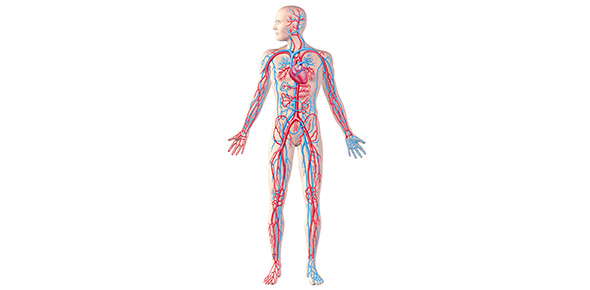Using A Haemocytometer

9 Questions | Total Attempts: 2867SettingsHUMAN BIOLOGY AS A quiz on how to use a haemocytometer

• 1.
A haemocytometer is a special counting chamber designed for counting _____ ____.
• 2.
What is the rule used when counting cells in a haemocytometer?
• A.

North East Rule

• B.

Counting Rule

• C.

South West Rule

• D.

North West Rule

• 3.
What squares are used when couting red blood cells?
• A.

The corner squares

• B.

The middle top square and middle bottom square

• C.

The 3 squares on the left and the 3 squares on the right

• D.

The middle square

• 4.
What is the dilution factor for red blood cells?
• A.

1:300

• B.

1:200

• C.

1:100

• D.

1:50

• 5.
What is the volume of one triple-lined square?
• A.

0.02mm³

• B.

0.002mm³

• C.

0.004mm³

• D.

0.04mm³

• 6.
What is the dilution factor for white blood cells?
• A.

1:100

• B.

1:50

• C.

1:20

• D.

1:10

• 7.
Where are white blood cells counted?
• A.

The middle square

• B.

The 3 left squares and 3 right squares

• C.

The squares in the corners

• D.

The 3 top squares and 3 bottom squares

• 8.
Why are several squares on the haemocytometer counted, rather than just one?
• A.

It is more representative

• B.

It is more fair

• C.

It makes the results more reliable

• D.

It is more valid

• 9.
When counting white blood cells, what is the volume of each square?
• A.

0.02mm³

• B.

0.01mm³

• C.

0.2mm³

• D.

0.1mm³

Related TopicsBack to top